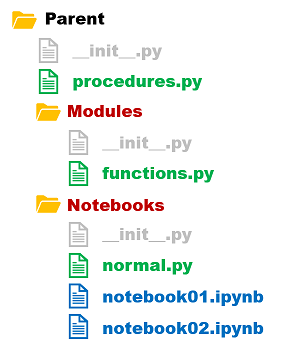# How to Import from Parent Folder in Python

Python programming language allows you to import various packages into your script, as per your requirement. Typically, developers install package using pip or easy_install utilities and import them into their script using import keyword. But sometimes you may need to import functions and variables from parent folder, or even an ancestor folder. In this article, we will learn how to import from parent folder in Python.

## How to Import from Parent Folder in Python

Let us say you have the following folder structure.

```a/
p.py

t.py
__init__.py
b/

s.py
__init__.py
c/
q.py
r.py
__init__.py```

Let us say you want to import function hello() into q.py from p.py. You can do this using relative imports.

`from ... import p.hello`

In the above code, we use three dots (…) to go two packages above and then import p.hello to our script. If you want to import everything from p.py to q.py you can modify the above command as shown.

`from ... import p`

If you want to import a variable TEST_VARIABLE you can do so using the following statement.

`from ... import p.TEST_VARIABLE`

You can use consecutive periods after from keyword to specify relative folder location for import. Since dot (.) means current folder, two consecutive dots(..) means parent folder, and three consecutive dots (…) means two folders up. Here is an example to demonstrate this.

```from . import r
from .. import s
from ... import t```

Please note, you need to add an empty __init__.py file to each folder from where you want to import functions and modules to another script. Please refer to our folder structure above.

In this article, we have learnt how to import functions, modules and variables from another folder, using relative imports in python.